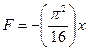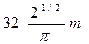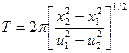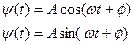Simple Harmonic Motion, iitjee questions on Simple Harmonic Motion, subjective questions on Simple Harmonic Motion"># Important subjective questions on Simple Harmonic Motion for JEE Main/Advanced

In this page we have Important subjective questions on Simple Harmonic Motion for JEE Main/Advanced . Hope you like them and do not forget to like , social share and comment at the end of the page.

Subjective Questions

Q-1:  A particle is situated at the end  of the one arm of a tuning fork passes  through its equilibrium position with a velocity of 2 ms-1.The amplitude is 10-3 m

a) Determine the frequency and time period of the tuning fork

b) Write the equations expressing its displacement and velocity as a function of time .Assume initial phase is zero

Q-2:  A 4 kg particle is moving along the x-axis under the action of the forceN

When t=2 sec the particle passes through the origin and when t=4 sec.its velocity is 4 m/s

a) Find the equation of the displacement

b) Show that the amplitude of motion isQ-3:  A particle is moving with SHM in a straight line, when the distance of the particle from the equilibrium position has the values x1 and x2, the corresponding velocities are u1 and u2. Show that time period of SHM isQ-4:  A cylinder of cross-sectional area S and length L is floating in a liquid of density ρ0. Density of the cylinder is ρ. If the cylinder is slightly depressed and released, then describe the motion of the cylinder

Q-5:

A particle executes SHM with amplitude A.If its starting point from rest  is

a)  ψ =+A

b) ψ =-A

c)  ψ =+A/2

Find the different values of the phase constant (Φ) for the equationsSolutions

Note to our visitors :-

Thanks for visiting our website.